# SBI PO 2022: Quantitative Aptitude Quiz- 10

## SBI PO Prelims 2022: Quantitative Aptitude

Quantitative aptitude is a quite important section of numerous banking exams, such as the IBPS, SBI, and RBI PO and Clerk exams. Many candidates face difficulty in solving Quantitative Aptitude Questions. Even if the portion is difficult, if you practice enough questions and learn the basic concepts, you can surely do well. So, here we bring to you the SBI PO 2022 Quantitative Aptitude Quiz. Candidates will be provided with a detailed explanation of each question in this SBI PO 2022 Quantitative Aptitude Quiz. This SBI PO 2022 Quantitative Aptitude Quiz is totally FREE for the Candidates. This SBI PO 2022 Quantitative Aptitude Quiz contains various questions ranging from simple to difficult levels. This SBI PO 2022 Quantitative Aptitude Quiz will assist the candidates with upgrading their preparation for upcoming examinations.

Directions (1-5): Given below is the pie chart which shows the percentage distribution of books of publisher ‘X’ sold by 7 different books store in year 2016. Table shows the ratio of books sold of publisher X to publisher Y in these seven book stores.
Some values are missing in the table. You have to calculate these values if required to answer the questions.1. What is the total number of books sold by store A and B together if books sold by store A for publisher Y is 100/3% more than that of publisher X and Books sold by store B for publisher X is 20% less than that of publisher Y.
(a) 22359
(b) 21257
(c) 20256
(d) 23244
(e) 22556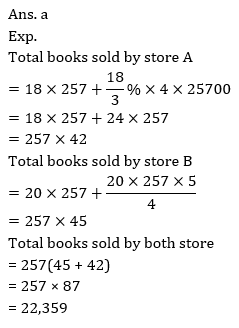2. What is the total number of books sold by store D if books sold of publisher Y in store D is 25% more than that of books sold by store D of publisher X
(a) 2520
(b) 4020
(c) 4626
(d) 4422
(e) 45283. Books sold by store E, F and G together of publisher X is what percent more or less than books sold by these store of publisher Y if books sold by store F of publisher Y is 100/11% more than that of books sold by F of publisher X.4. If in year 2017 total books sold by store E is increased by 100/3% over previous year and ratio of books sold of publisher X and Y by store E in 2017 is 11 : 13 then books sold by store E of publisher X in 2016 is what percent more or less than that of books sold of publisher X by store E in 2017.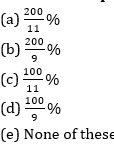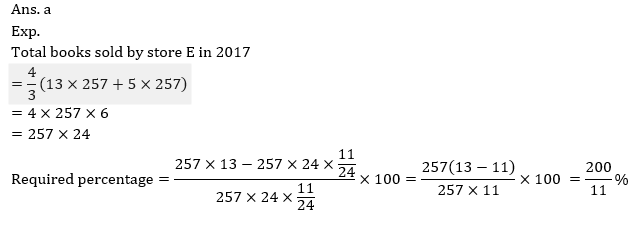5. Average of books of publisher X sold by store B and C together is what percent more or less than that of average of books of publisher Y sold by store E and G together?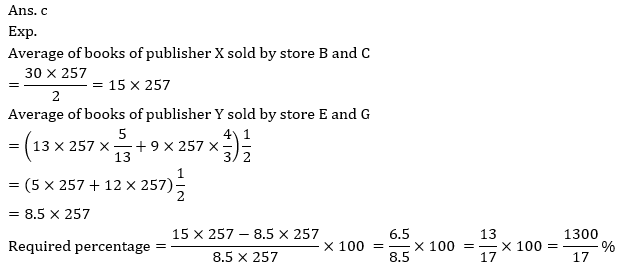6. Population of city P in 2004 is 52000 and the ratio between the number of male to the number of female is 7 : 6.Male population of city P increase by 100/7% in 2005 and by 25/2% in 2006. At the end of 2006 total population of city P becomes 475/13% more than the total population of city P in 2004. If female population is increased by 50/3% in 2005, then find by what percent the female population increases in 2006.
(a) 17.5%
(b) 10%
(c) 15%
(d) 25%
(e) 20%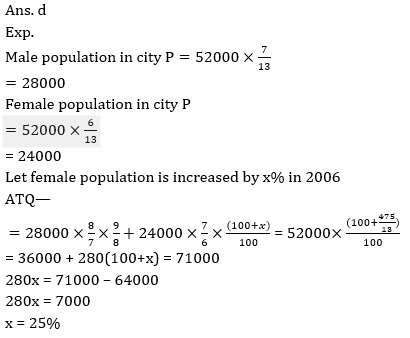7. The distance covered by a car in T hours is 120 km less than the distance covered by train in the same duration, if the speed of train is 100/3% more than the speed of car, which is 72 km/hr and the speed of an aircraft is 87½ % more than speed of train. Find how much distance will be covered by aircraft in (T –1/2) hour?
(a) 640 km
(b) 810 km
(c) 720 km
(d) 960km
(e) 1280km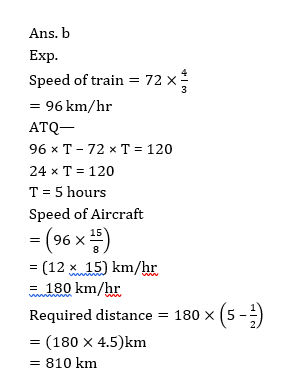8. Vinay invested Rs. 1700 in a scheme ‘A’ for 2 years which offered S.I. annually at the rate of R% and gets total interest of Rs. 544. If he reinvested the total amount (principle + interest) received from scheme A in an another scheme B for two years, which offered CI annually at the rate of (R + 4) %, then find the total interest received by Vinay from the scheme B ?
(a) 967.36Rs.
(b) 987.36Rs.
(c) 997.36Rs.
(d) 990.89Rs.
(e) 988.88Rs.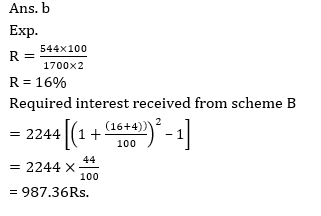9. Three containers having capacities in the ratio of 5 : 8 : 3 contain mixture of milk and water in the ratio 3 : 1, 3 : 2 and 2 : 1 respectively. If all three containers are poured in a big container, then find the ratio of milk to water in the final mixture?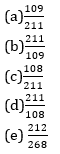10. If a shopkeeper increased the marked price of an article by 60% and gives three successive discounts of 25/2%,100/7% and 20% on the new marked price of the article then he makes overall profit of 20%. If he gives only two successive discounts of 25/2% and 20% on new M.P then he makes overall profit of Rs. 800. Find the cost price of the article?
(a) Rs. 2000
(b) Rs.2400
(c) Rs. 2800
(d) Rs. 3000
(e) Rs. 3200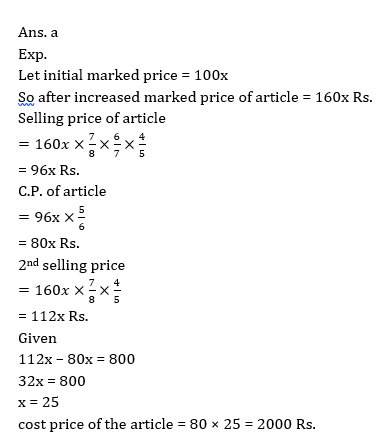#### Attempt Quantitative Aptitude Topic Wise Online Test Series

Recommended PDF’s for:

#### Most important PDF’s for Bank, SSC, Railway and Other Government Exam : Download PDF Now

AATMA-NIRBHAR Series- Static GK/Awareness Practice Ebook PDF Get PDF here
The Banking Awareness 500 MCQs E-book| Bilingual (Hindi + English) Get PDF here
AATMA-NIRBHAR Series- Banking Awareness Practice Ebook PDF Get PDF here
Computer Awareness Capsule 2.O Get PDF here
AATMA-NIRBHAR Series Quantitative Aptitude Topic-Wise PDF Get PDF here
AATMA-NIRBHAR Series Reasoning Topic-Wise PDF Get PDF Here
Memory Based Puzzle E-book | 2016-19 Exams Covered Get PDF here
Caselet Data Interpretation 200 Questions Get PDF here
Puzzle & Seating Arrangement E-Book for BANK PO MAINS (Vol-1) Get PDF here
ARITHMETIC DATA INTERPRETATION 2.O E-book Get PDF here

3